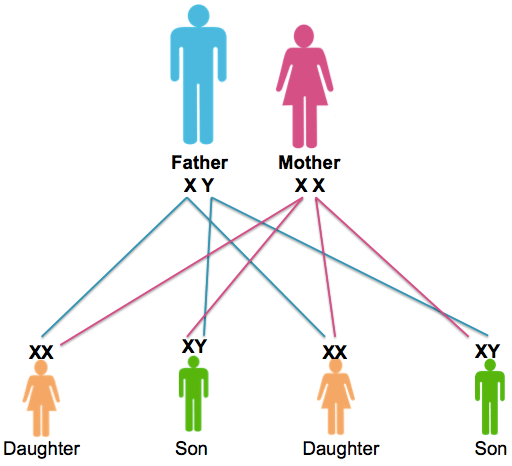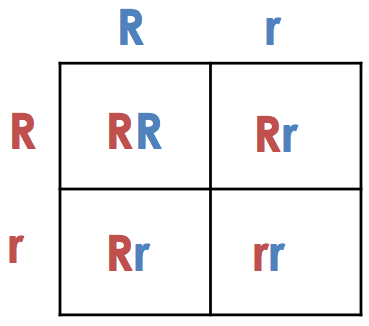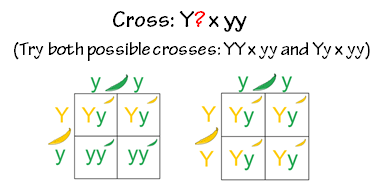# Using Punnett Squares to Predict Offspring

How do Punnett squares help us to make valid predictions about offspring?

In the last lesson, we discussed traits and how we represent those when talking about organisms. You should have a good understanding of what phenotype and genotype mean, as well as what letter combinations make a genotype homozygous or heterozygous.

In this lesson, we are going to learn more about how we can predict the kind of offspring that will result from matings. Scientists would make predictions based on observations of those traits, and then test out their ideas about the inheritance of a genetic trait by crossing the organisms in question and examining the offspring.

## Understanding Probability

To understand genetics and make predictions, one must understand probabilities.

The traits that are passed from parents to offspring are determined by the combination of genes (genotype) that are in the gametes. Which allele is passed on by a parent is a random event in nature. The probability of a particular event occurring is the fraction of outcomes in which that event occurs. Probability is the likely outcome a given event will occur from random chance.

A coin toss is a random (but fair) decision.

In football or otherwise, each time a coin is flipped, there is a 50% chance of heads and 50% chance of tails. Likewise, the chance of inheriting one of two alleles from a parent is also 50% or 1/2. Examine the picture below that shows how sex chromosomes are inherited from parents.Remember that females have two X chromosomes (XX), and males have an X and a Y chromosome (XY). Women donate only X’s to their offspring in their egg, but males may donate either their X or Y chromosome in their sperm. This means there is a 50/50 chance that a child will receive an X from the father. Or a 50/50 chance they will receive a Y.Stop and Think: Which parent determine the sex of a baby? Is it the father or the mother? (Answer: The father. The father contributes either an X or a Y chromosome. The mother only contributes an X.)

Look at the diagram above again. If the probability of having a boy is 1/2, then the probability of having two boys at the same time or two boys consecutively is 1/2 x 1/2 = 1/4, or 25%. Each event (birth) has its own probability so the probability that the next child will be a boy is still 1/2.Stop and Think: Look at the family above. Mom and dad already have three girls in their family. What is the probability that the fourth child with be a boy?  (answer: every child has the same 1/2, 50% chance)

What is the probability of rolling two fives with a pair of dice? For each die there is a 1/6 chance of getting a five. To get two at the same time, multiply the probability of getting a five times the probability of getting another five: 1/6 x 1/6 = 1/36 chance for getting two fives.

## What is a Punnett Square?

Punnett Square (so named after it’s creator, Reginald C. Punnett) is a chart drawn to determine the probable results of a genetic cross. It will show you every possible combination of offspring that result from a cross. Therefore, a Punnett square is a prediction that estimates what we should see in nature.

### How does a Punnett Square Work?

To be able to draw a Punnett Square, you must know the genotype of both parents.

The genes of the parents represented by assigned letters are written on the left-hand side and the top of the Punnett Square. Alleles of each gene are separated above each column (on the top) or into rows (on the bottom).For example if the letter Y represents the gene for pea pod color where yellow is dominant to green. One parent is heterozygous Yy and the other is homozygous yy, the completed Punnett Square would look like the one on the right.

A completed Punnett square gives the probable outcome of a given cross. In this case, the probability of each phenotype (green vs. yellow) is 50%. The probability of offspring with genotype Yy is 50%, as the probability of genotype yy. We can write these offspring probabilities as ratios to simplify our results:

Phenotype Ratio : 2 yellow: 2 green

Genotype Ratio : 2 Yy: 2yy

## Using Punnett Squares to Solve Genetics Problems: Step by Step

In this section, follow the tutorial so that you can learn how to set up your own Punnett squares to solve genetics problems. You will use this skill for the rest of the module, so take your time.

Sample Problem #1: In guinea pigs, straight hair (H) is dominant to curly hair (h). What would be the results of a mating between a curly-haired guinea pig and heterozygous straight-haired guinea pig?

A printable version of this tutorial is found in the sidebar.

Sample Problem #2: In peas, round seeds (R) are dominant to wrinkled seeds (r). Show the possible offspring for a cross between two pea plants that are both heterozygous for this trait.

Step 1: Identify the cross: Rr x Rr

Step 2:What gametes can each parent make? Each Rr parent makes R and r gametes.

Steps 3 and 4: Set up and solve your cross as follows:What genotypes are predicted by this square? (answer: One RR (homozygous dominant), Two Rr (heterozygous) and One rr (homozygous recessive))

What phenotypes are predicted by this square? (answer: Three red, one white)

Write the genotype and phenotype ratios for the cross: (answer: Genotype Ratio: 1RR: 2Rr: 1rr; Phenotype Ratio: 3 Red: 1 White)

Let’s revisit Mendel’s original experiment now that we understand how to use Punnett squares to predict mating outcomes. Click through the interactive to refine your understanding about what Mendel saw.

## The Testcross: A Punnett Square That Solves a “Dominant” MysterySometimes scientists need to find out whether or not an organism’s genotype is pure (homozygous) dominant or hybrid (heterozygous) dominant for a trait. A testcross is performed to solve this mystery.

testcross involves crossing the unknown organism with a an organism that is known to be homozygous recessive. If any of the offspring are homozygous recessive and show the recessive phenotype, then the unknown had to be heterozygous.

Look below at the two possible outcomes of a testcross. In both, one of the individuals is a known recessive (green pods: yy).

1. If the mystery plant is a heterozygote (Yy) then you will see recessive phenotypes in the offspring.
2. If the mystery plant is a homozygote (YY) then you will see all dominant phenotypes in the offspring.## Monohybrid v. Dihybrid Crosses

So far, all of the Punnett square problems you have been solving have involved only one trait, otherwise called a monohybrid cross. A dihybrid cross, on the other hand, is a cross where two pairs of contrasting genes (such as flower color and seed color) are examined at the same time.

A dihybrid cross would result in a Punnett square that is usually larger because more gamete types are possible. Each gamete (row or column) would have two letters, and each offspring box would have four letters.

A cat that has a short tail (Ss) and Brown fur (Bb) can be crossed with another cat that has the same genotype (SsBb). To do this cross, a 16 box Punnett square must be used.

SsBb x SsBb

To determine what alleles to place on the outside of the squares, recall how meiosis would combine Ss and Bb…only one S or s and one B or b in each gamete. There are four possible combinations of gametes for either parent (since they have the same genotype, SsBb):

SB, sB, Sb and sb

Take a look at the Punnett square below that shows the results of this same cross. The traits are long tail (s), short tail (S), brown fur (B) and white fur (b).Whenever two individuals that are heterozygous for both traits are involved in a Punnett square, we see four different phenotype groups with a typical 9:3:3:1 phenotype ratio.

Stop and Think: Look again at the offspring from this cross and see if you can separate out each of the four different phenotype groups.

Phenotype ratio: 9_____; 3_____; 3_____; 1_____  (answers: 9 short tail, brown fur (purple) — 3 short tail, white fur (pink) — 3 long tail, brown fur (blue) — 1 long tail, white fur (green) )

(source)